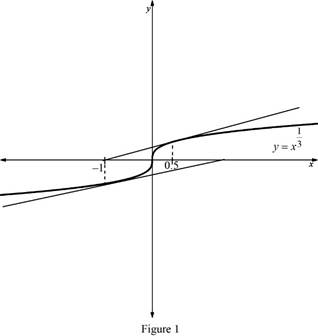# The Newton’s method fails for the given equation with any approximation x 1 ≠ 0 with sketch the graph.### Single Variable Calculus: Concepts...

4th Edition
James Stewart
Publisher: Cengage Learning
ISBN: 9781337687805### Single Variable Calculus: Concepts...

4th Edition
James Stewart
Publisher: Cengage Learning
ISBN: 9781337687805

#### Solutions

Chapter 4.7, Problem 27E
To determine

## To find: The Newton’s method fails for the given equation with any approximation x1≠0 with sketch the graph.

Expert Solution

### Explanation of Solution

Given:

The equation is x13=0.

The initial approximation is x1=0.5.

Result Used:

The Newton’s method is xn+1=xnf(xn)f(xn).

Graph:From the Figure 1, it is observed that the value gets doubled.

Calculation:

Let f(x)=x13, then f(x)=0 by the given condition.

Calculate the derivative of f(x).

Let f(x)=df(x)dx.

f(x)=d(x13)dx=13x23

Substitute the value of f(x) and f(x) in the above mentioned result to obtain xn+1.

xn+1=xnxn1313xn23=xn3xn=2xn

Set n=1, x1=0.5 and obtain x2 from the above expression.

x2=2×0.5=1

Set n=2, x2=1 and obtain x3 from the above expression.

x3=2×(1)=2

Similarly, proceed in the above manner.

Observation:

From the above calculation, each successive approximation gives the value twice than previous value.

Thus, if we consider the sequence of the Newton’s approximation fails to converge to the root 0. Therefore, the Newton’s method fails.

### Have a homework question?

Subscribe to bartleby learn! Ask subject matter experts 30 homework questions each month. Plus, you’ll have access to millions of step-by-step textbook answers!